Get instant live expert help with Excel or Google Sheets“My Excelchat expert helped me in less than 20 minutes, saving me what would have been 5 hours of work!”

#### Post your problem and you’ll get Expert help in seconds.

Your message must be at least 40 characters
Our professional Expert are available now. Your privacy is guaranteed.

# Count cells equal to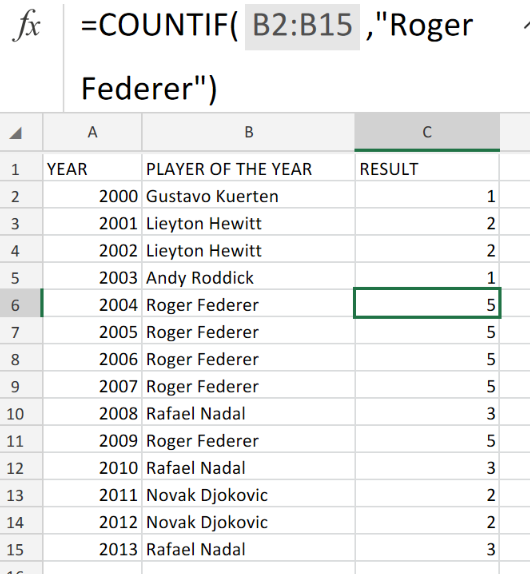Figure 1. of the Count Cells Equal To Function in Excel.

Let’s say we have a range of data values in our Excel sheet, and we want to count how many cells meet a specific criteria. This can be acheived through the COUNTIF function. This tutorial will step through the process of counting cells equal to a specific value.

## Generic Formula

`=COUNTIF(range,value)`

## How to use the Excel COUNTIF Function.

The COUNTIF Function in Excel is fully automated. It is designed to automatically count the amount of cells within a range which matches with our specified criteria.

We can achieve this by following three simple steps;

1. Arrange the total data values in our Excel sheet by column.

In our example illustrated below, we have 3 column – YEAR, PLAYER OF THE YEAR (which contain the year of the annual ceremony and the name of the players) and the RESULT column.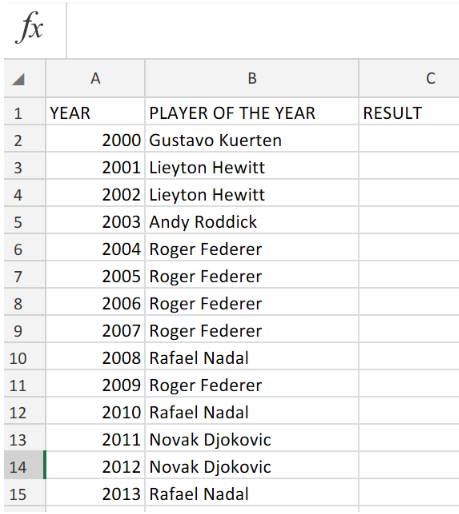Figure 2. of Data Values in Excel.

1. Input the following formula syntax into cell C6 of our worksheet;

`=COUNTIF(B2:B15,"Roger Federer")`

This will automatically count the number of times that particular player’s name occurs in our list of data values.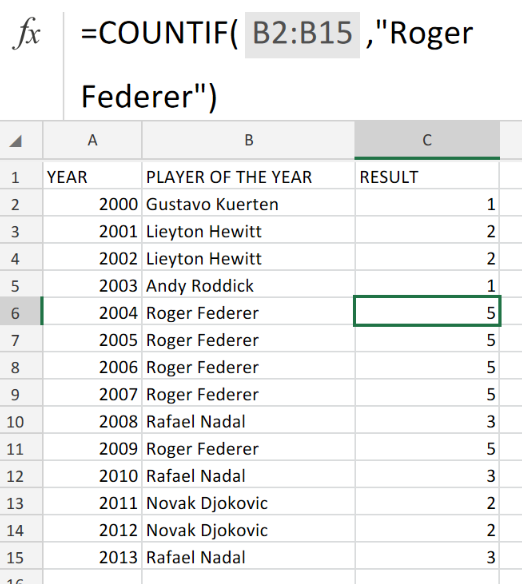Figure 3. of the Count Cells Equal To Function in Excel.

Since the name “Roger Federer” occurs in five separate years in our list of data values, the Excel COUNTIF operation syntax returns the result as 5.

1. Modify and Copy the formula syntax in cell C6 into the other cells in the RESULT column of our worksheet to achieve the desired results.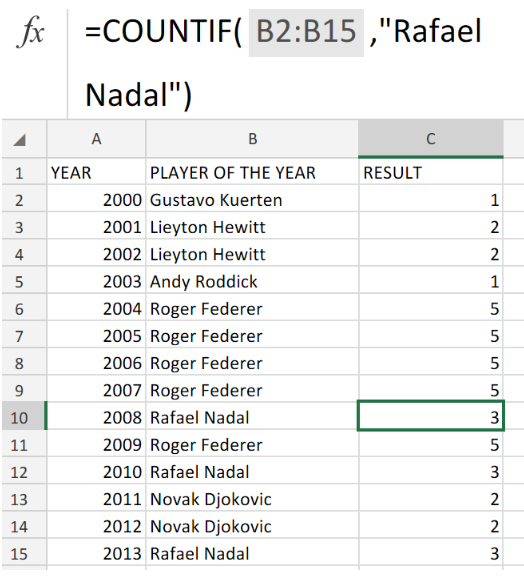Figure 4. of the Count Cells Equal To Function in Excel.

## Note

• For the range (in the formula syntax) in our example, we use B2:B15.
• For the value (in the formula syntax) in our example, we use the player’s name.
• When our data values are in the form of text, they must be enclosed in double quotes     ( “ “ ).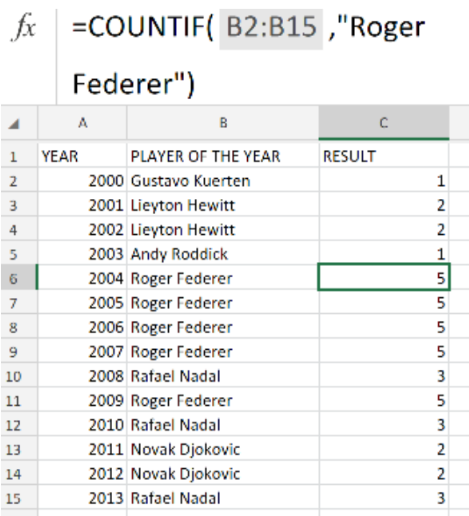Figure 5. of Final Result.

## Instant Connection to an Expert through our Excelchat Service

Our live Excelchat Service is here for you. We have Excel Experts available 24/7 to answer any Excel questions you may have. Guaranteed connection within 30 seconds and a customized solution for you within 20 minutes

### Did this post not answer your question? Get a solution from connecting with the expert.Another blog reader asked this question today on Excelchat:
Solution examplesI need a formula to count the number of rows that contain at least one of two possible phrases, but not give me the total number of instances that both phrases occur overall.
Solved by E. B. in 33 minsi have a table with Dates, First names , Last names and i want to count how many times a name occurs, but if a name occurs more than once on a particular date i need to only count it once. this is on excel and not google sheets
Solved by F. H. in 31 minsNeed a formula that counts (1,2,3,4 ect..in cell M21) a range of cells, N4:N20, which are annual premium values. But I only want to add a sale count if the average monthly premium is above \$30 per sale. So if someone has 4 sales but they don't equal a total of \$120/mth (\$30 x 4 = \$120)... they would only get a count of 3 sales.
Solved by S. E. in 60 minsHello, I am trying to create a total color column? Can anyone help?
Solved by I. A. in 60 minsAny rep that has orders over 500 and occurrences at zero for each month, for a 6 month period. I need a sum
Solved by C. E. in 60 mins## Subscribe to Excelchat.coAnother blog reader asked this question today on Excelchat: